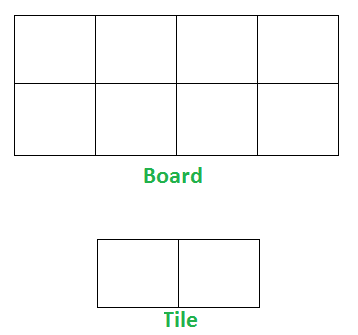# Tiling Problem

• Difficulty Level : Easy
• Last Updated : 24 May, 2022

Given a “2 x n” board and tiles of size “2 x 1”, count the number of ways to tile the given board using the 2 x 1 tiles. A tile can either be placed horizontally i.e., as a 1 x 2 tile or vertically i.e., as 2 x 1 tile.

Examples:

Input: n = 4

Output: 5

Explanation:

For a 2 x 4 board, there are 5 ways

• All 4 vertical (1 way)
• All 4 horizontal (1 way)
• 2 vertical and 2 horizontal (3 ways)

Input: n = 3

Output: 3

Explanation:

We need 3 tiles to tile the board of size  2 x 3.

We can tile the board using following ways

• Place all 3 tiles vertically.
• Place 1 tile vertically and remaining 2 tiles horizontally (2 ways)Implementation –

Let “count(n)” be the count of ways to place tiles on a “2 x n” grid, we have following two ways to place first tile.
1) If we place first tile vertically, the problem reduces to “count(n-1)”
2) If we place first tile horizontally, we have to place second tile also horizontally. So the problem reduces to “count(n-2)”
Therefore, count(n) can be written as below.

```   count(n) = n if n = 1 or n = 2
count(n) = count(n-1) + count(n-2)```

Here’s the code for the above approach:

## C++

 `// C++ program to count the``// no. of ways to place 2*1 size``// tiles in 2*n size board.``#include ``using` `namespace` `std;` `int` `getNoOfWays(``int` `n)``{``    ``// Base case``    ``if` `(n <= 2)``      ``return` `n;` `    ``return` `getNoOfWays(n - 1) + getNoOfWays(n - 2);``}` `// Driver Function``int` `main()``{``    ``cout << getNoOfWays(4) << endl;``    ``cout << getNoOfWays(3);``    ``return` `0;``}`

## Java

 `/* Java program to count the`` ``no of ways to place 2*1 size`` ``tiles in 2*n size board. */``import` `java.io.*;` `class` `GFG {``  ``static` `int` `getNoOfWays(``int` `n)``  ``{` `    ``// Base case``    ``if` `(n <= ``2``) {``      ``return` `n;``    ``}``    ``return` `getNoOfWays(n - ``1``) + getNoOfWays(n - ``2``);``  ``}` `  ``// Driver Function``  ``public` `static` `void` `main(String[] args)``  ``{``    ``System.out.println(getNoOfWays(``4``));``    ``System.out.println(getNoOfWays(``3``));``  ``}``}` `// This code is contributed by ashwinaditya21.`

## Python3

 `# Python3 program to count the``# no. of ways to place 2*1 size``# tiles in 2*n size board.``def` `getNoOfWays(n):``  ` `    ``# Base case``    ``if` `n <``=` `2``:``        ``return` `n` `    ``return` `getNoOfWays(n ``-` `1``) ``+` `getNoOfWays(n ``-` `2``)` `# Driver Code``print``(getNoOfWays(``4``))``print``(getNoOfWays(``3``))` `# This code is contributed by Kevin Joshi`

## C#

 `// C# program to implement``// the above approach``using` `System;` `class` `GFG``{``    ` `static` `int` `getNoOfWays(``int` `n)``  ``{`` ` `    ``// Base case``    ``if` `(n <= 2) {``      ``return` `n;``    ``}``    ``return` `getNoOfWays(n - 1) + getNoOfWays(n - 2);``  ``}` `// Driver Code``public` `static` `void` `Main()``{``    ``Console.WriteLine(getNoOfWays(4));``    ``Console.WriteLine(getNoOfWays(3));``}``}` `// This code is contributed by code_hunt.`

## Javascript

 ``

Output:

```5
3```

The above recurrence is nothing but Fibonacci Number expression. We can find n’th Fibonacci number in O(Log n) time, see below for all method to find n’th Fibonacci Number.
https://youtu.be/NyICqRtePVs
https://youtu.be/U9ylW7NsHlI
Different methods for n’th Fibonacci Number
Count the number of ways to tile the floor of size n x m using 1 x m size tiles